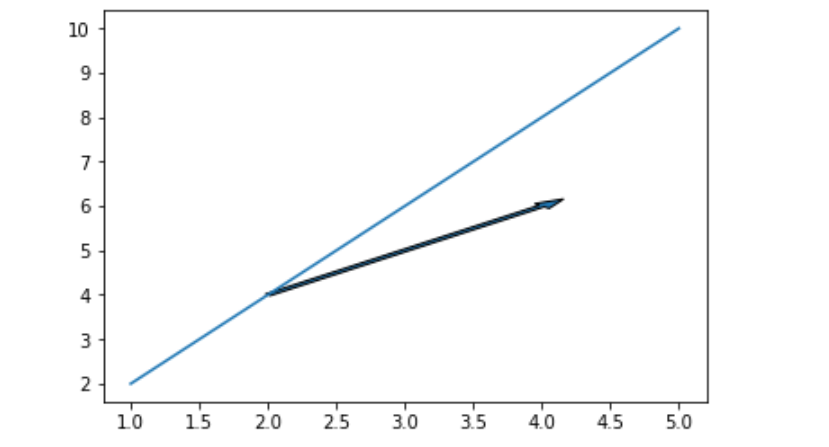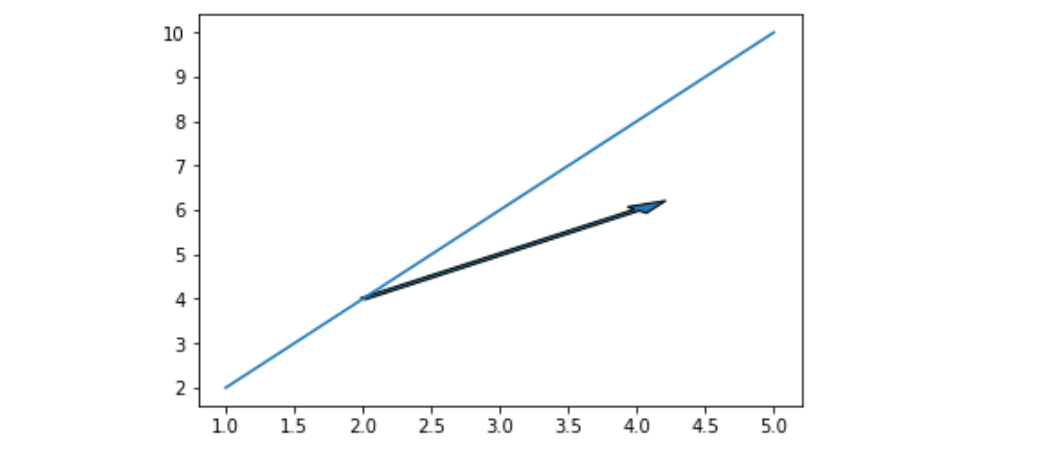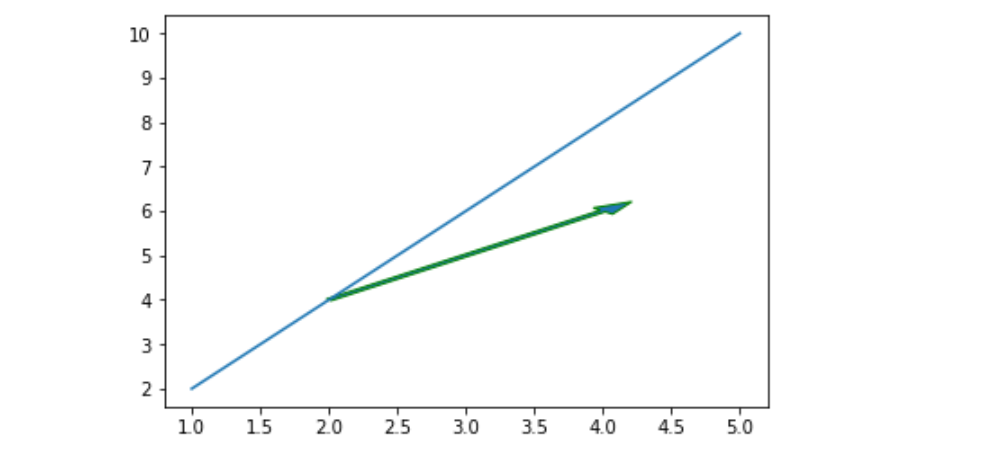# matplotlib.pyplot.arrow() in Python

Matplotlib is a very powerful plotting library useful for those working with Python and NumPy. And for making statistical interference, it becomes very necessary to visualize our data and Matplotlib is the tool that can be very helpful for this purpose. It provides MATLAB like interface only difference is that it uses Python and is open source.

## matplotlib.pyplot.arrow()

This function adds the arrow to the graph based on the coordinates passed to it.

Syntax: matplotlib.pyplot.arrow(x, y, dx, dy, **kwargs)
Parameters:
x, y: The x and y coordinates of the arrow base.
dx, dy: The length of the arrow along x and y direction.
**kwargs: Optional arguments that helps in adding properties to arrow, like
adding color to arrow, changing width of arrow

Example #1

## Python3

 `import` `matplotlib.pyplot as plt`     `# Initializing values ` `# of x and y` `x ``=``[``1``, ``2``, ``3``, ``4``, ``5``]` `y ``=``[``2``, ``4``, ``6``, ``8``, ``10``]`   `# Plotting the graph` `plt.plot(x, y)`   `# Adding an arrow to graph starting ` `# from the base (2, 4) and with the ` `# length of 2 units from both x and y` `# And setting the width of arrow for` `# better visualization` `plt.arrow(``2``, ``4``, ``2``, ``2``, width ``=` `0.05``)`   `# Showing the graph` `plt.show()`

Output:Example 2#

## Python3

 `import` `matplotlib.pyplot as plt`     `x ``=``[``1``, ``2``, ``3``, ``4``, ``5``]` `y ``=``[``2``, ``4``, ``6``, ``8``, ``10``]`   `plt.plot(x, y)`   `# Increasing head_width of` `# the arrow by setting ` `# head_width parameter` `plt.arrow(``2``, ``4``, ``2``, ``2``, ` `          ``head_width ``=` `0.2``, ` `          ``width ``=` `0.05``)`   `plt.show()`

Output:Example #3

## Python3

 `import` `matplotlib.pyplot as plt`     `x ``=``[``1``, ``2``, ``3``, ``4``, ``5``]` `y ``=``[``2``, ``4``, ``6``, ``8``, ``10``]` `plt.plot(x, y)`   `# changing the edge color` `# to green` `plt.arrow(``2``, ``4``, ``2``, ``2``, ` `          ``head_width ``=` `0.2``,` `          ``width ``=` `0.05``,` `          ``ec ``=``'green'``)`   `plt.show()`

Output:Whether you're preparing for your first job interview or aiming to upskill in this ever-evolving tech landscape, GeeksforGeeks Courses are your key to success. We provide top-quality content at affordable prices, all geared towards accelerating your growth in a time-bound manner. Join the millions we've already empowered, and we're here to do the same for you. Don't miss out - check it out now!

Previous
Next